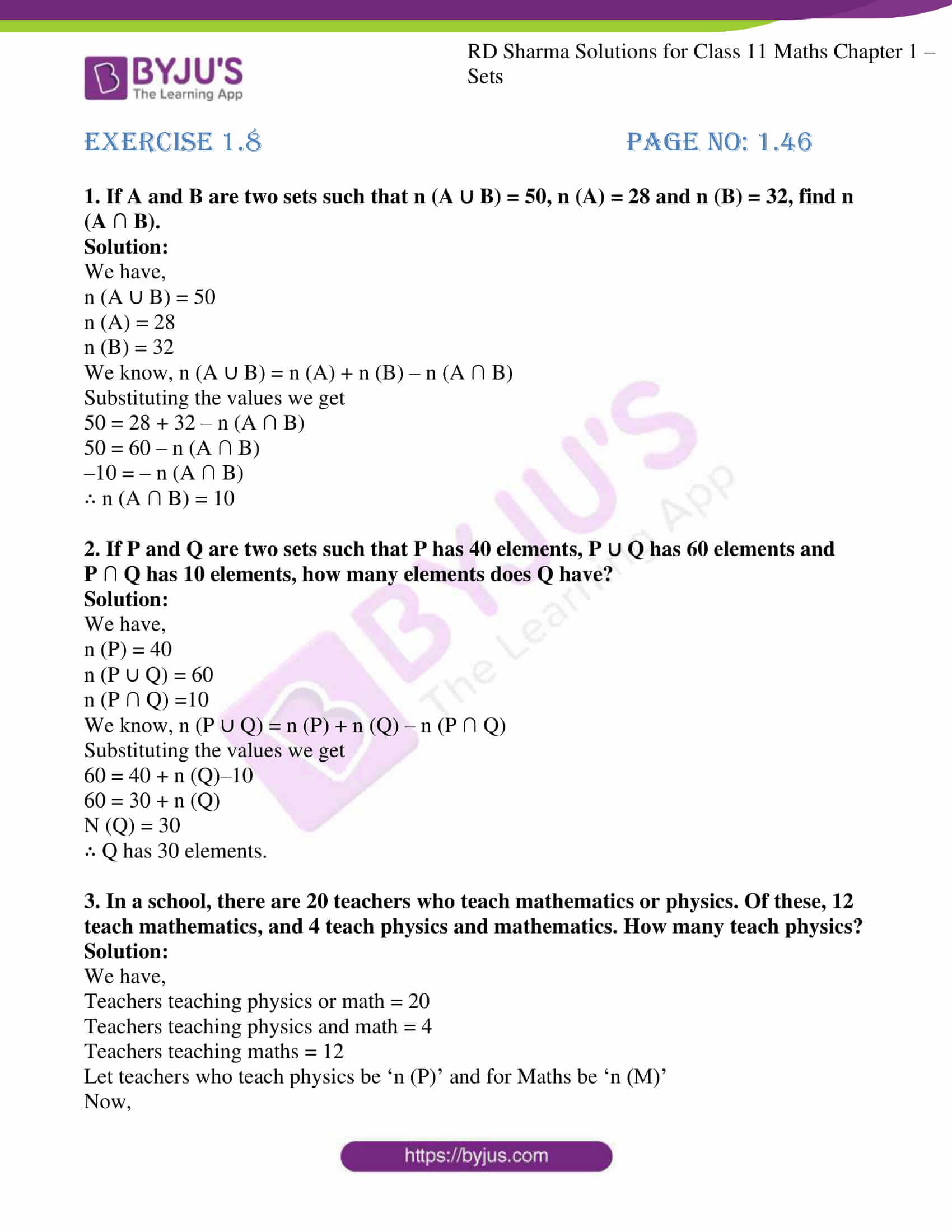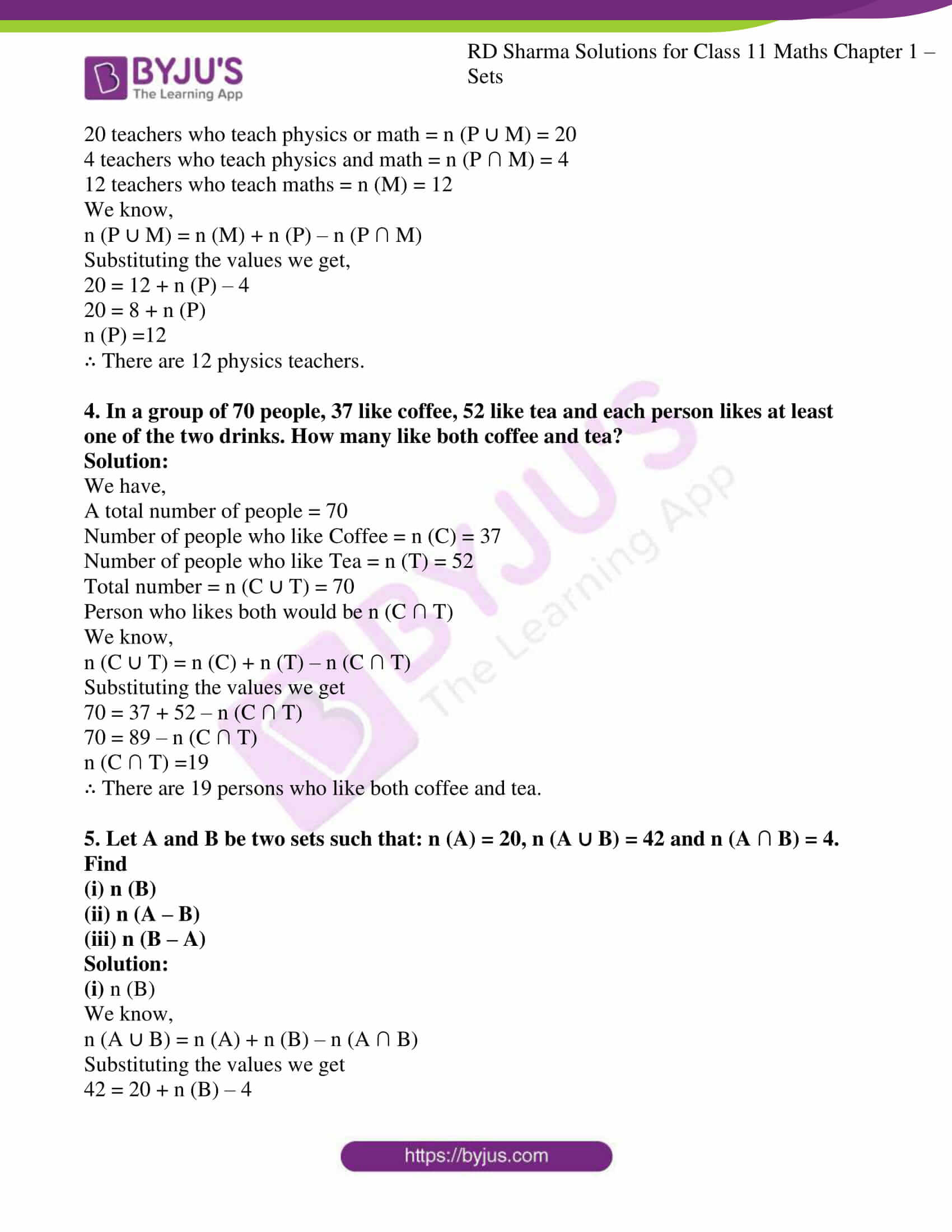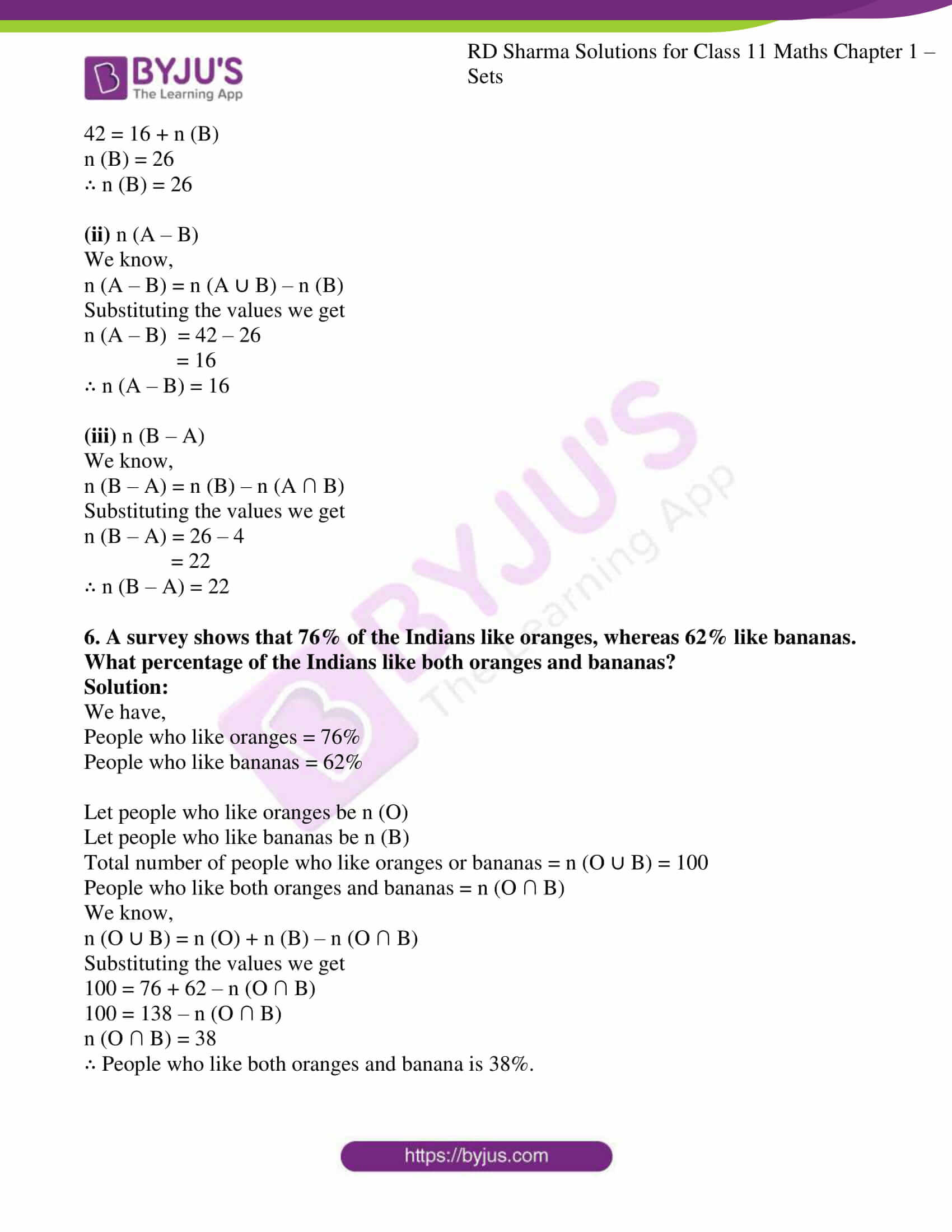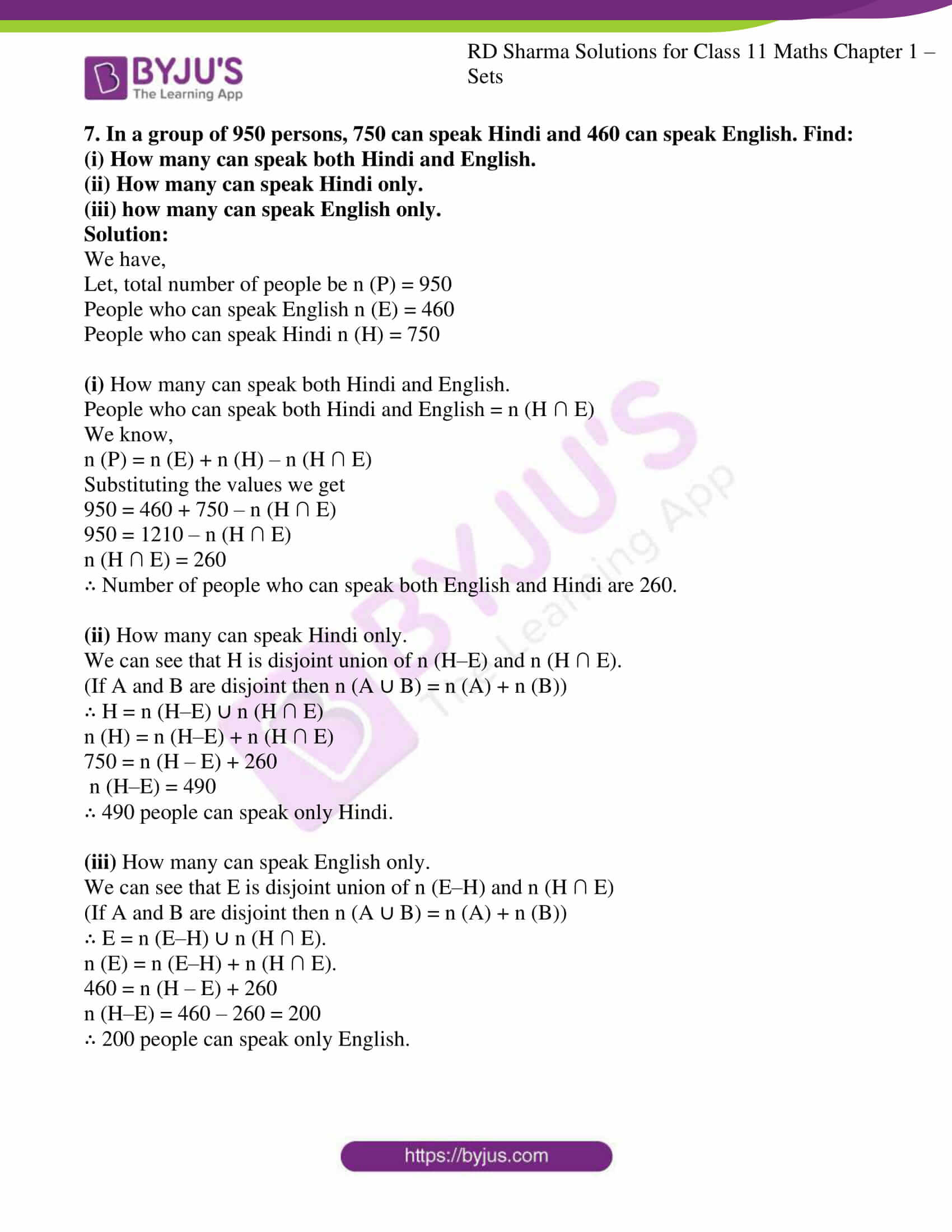# RD Sharma Solutions for Class 11 Chapter 1 - Sets Exercise 1.8

In Exercise 1.8, we shall study some important results on a number of elements in sets. Students can refer to RD Sharma Class 11 solutions which are prepared by our expert faculty team to help students clarify their doubts pertaining to this chapter. On regular practice, students can speed up the method of solving problems by using short-cut tips to secure high marks in their board examination. Students can easily download the pdf from the links given below.

## Download the Pdf of RD Sharma Solutions for Class 11 Maths Exercise 1.8 Chapter 1 – Sets### Access answers to RD Sharma Solutions for Class 11 Maths Exercise 1.8 Chapter 1 – Sets

1. If A and B are two sets such that n (A ∪ B) = 50, n (A) = 28 and n (B) = 32, find n (A ∩ B).

Solution:

We have,

n (A ∪ B) = 50

n (A) = 28

n (B) = 32

We know, n (A ∪ B) = n (A) + n (B) – n (A ∩ B)

Substituting the values we get

50 = 28 + 32 – n (A ∩ B)

50 = 60 – n (A ∩ B)

–10 = – n (A ∩ B)

∴ n (A ∩ B) = 10

2. If P and Q are two sets such that P has 40 elements, P ∪ Q has 60 elements and P ∩ Q has 10 elements, how many elements does Q have?

Solution:

We have,

n (P) = 40

n (P ∪ Q) = 60

n (P ∩ Q) =10

We know, n (P ∪ Q) = n (P) + n (Q) – n (P ∩ Q)

Substituting the values we get

60 = 40 + n (Q)–10

60 = 30 + n (Q)

N (Q) = 30

∴ Q has 30 elements.

3. In a school, there are 20 teachers who teach mathematics or physics. Of these, 12 teach mathematics, and 4 teach physics and mathematics. How many teach physics?

Solution:

We have,

Teachers teaching physics or math = 20

Teachers teaching physics and math = 4

Teachers teaching maths = 12

Let teachers who teach physics be ‘n (P)’ and for Maths be ‘n (M)’

Now,

20 teachers who teach physics or math = n (P ∪ M) = 20

4 teachers who teach physics and math = n (P ∩ M) = 4

12 teachers who teach maths = n (M) = 12

We know,

n (P ∪ M) = n (M) + n (P) – n (P ∩ M)

Substituting the values we get,

20 = 12 + n (P) – 4

20 = 8 + n (P)

n (P) =12

∴ There are 12 physics teachers.

4. In a group of 70 people, 37 like coffee, 52 like tea and each person likes at least one of the two drinks. How many like both coffee and tea?

Solution:

We have,

A total number of people = 70

Number of people who like Coffee = n (C) = 37

Number of people who like Tea = n (T) = 52

Total number = n (C ∪ T) = 70

Person who likes both would be n (C ∩ T)

We know,

n (C ∪ T) = n (C) + n (T) – n (C ∩ T)

Substituting the values we get

70 = 37 + 52 – n (C ∩ T)

70 = 89 – n (C ∩ T)

n (C ∩ T) =19

∴ There are 19 persons who like both coffee and tea.

5. Let A and B be two sets such that: n (A) = 20, n (A ∪ B) = 42 and n (A ∩ B) = 4. Find

(i) n (B)

(ii) n (A – B)

(iii) n (B – A)

Solution:

(i) n (B)

We know,

n (A ∪ B) = n (A) + n (B) – n (A ∩ B)

Substituting the values we get

42 = 20 + n (B) – 4

42 = 16 + n (B)

n (B) = 26

∴ n (B) = 26

(ii) n (A – B)

We know,

n (A – B) = n (A ∪ B) – n (B)

Substituting the values we get

n (A – B) = 42 – 26

= 16

∴ n (A – B) = 16

(iii) n (B – A)

We know,

n (B – A) = n (B) – n (A ∩ B)

Substituting the values we get

n (B – A) = 26 – 4

= 22

∴ n (B – A) = 22

6. A survey shows that 76% of the Indians like oranges, whereas 62% like bananas. What percentage of the Indians like both oranges and bananas?

Solution:

We have,

People who like oranges = 76%

People who like bananas = 62%

Let people who like oranges be n (O)

Let people who like bananas be n (B)

Total number of people who like oranges or bananas = n (O ∪ B) = 100

People who like both oranges and bananas = n (O ∩ B)

We know,

n (O ∪ B) = n (O) + n (B) – n (O ∩ B)

Substituting the values we get

100 = 76 + 62 – n (O ∩ B)

100 = 138 – n (O ∩ B)

n (O ∩ B) = 38

∴ People who like both oranges and banana is 38%.

7. In a group of 950 persons, 750 can speak Hindi and 460 can speak English. Find:
(i) How many can speak both Hindi and English.
(ii) How many can speak Hindi only.
(iii) how many can speak English only.

Solution:

We have,

Let, total number of people be n (P) = 950

People who can speak English n (E) = 460

People who can speak Hindi n (H) = 750

(i) How many can speak both Hindi and English.

People who can speak both Hindi and English = n (H ∩ E)

We know,

n (P) = n (E) + n (H) – n (H ∩ E)

Substituting the values we get

950 = 460 + 750 – n (H ∩ E)

950 = 1210 – n (H ∩ E)

n (H ∩ E) = 260

∴ Number of people who can speak both English and Hindi are 260.

(ii) How many can speak Hindi only.

We can see that H is disjoint union of n (H–E) and n (H ∩ E).

(If A and B are disjoint then n (A ∪ B) = n (A) + n (B))

∴ H = n (H–E) ∪ n (H ∩ E)

n (H) = n (H–E) + n (H ∩ E)

750 = n (H – E) + 260

n (H–E) = 490

∴ 490 people can speak only Hindi.

(iii) How many can speak English only.

We can see that E is disjoint union of n (E–H) and n (H ∩ E)

(If A and B are disjoint then n (A ∪ B) = n (A) + n (B))

∴ E = n (E–H) ∪ n (H ∩ E).

n (E) = n (E–H) + n (H ∩ E).

460 = n (H – E) + 260

n (H–E) = 460 – 260 = 200

∴ 200 people can speak only English.